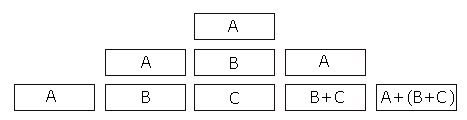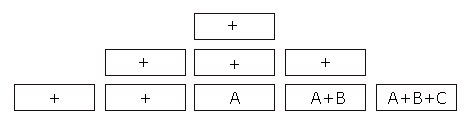## Prefix, Infix, Postfix

### What are prefix, infix and postfix?

Prefix, infix and postfix are methods of reading numerical expressions. The pre, in, and post all refer to the position of our operators in relation to the operands. We use infix, which means that when we take A times B, we write it as A*B. In postfix, it would be written as AB* and in infix it would be written as *AB. Postfix is most common on WYSE tests, and is commonly referred to as revere polish notation.

### Infix: It’s easy

Infix needs no explanation. We use infix every day and it’s what we’re all used to.

### Postfix/Reverse Polish Notation

Obviously things get more complicated than just AB*. The best way to use postfix is to use a stack and add to the stack (if you don’t know what I mean, just keep going). Let’s take the postfix expression ABC++. Below is the stack method for determining the infix of this — just be patient and I’ll explain each process.Each digit is entered into the stack, the oldest digit moving up and the newest are at the bottom. Once you get an operator (+,-,*,/,etc.) you take the bottom two stack items and put them together. So walking through the stack, the first three digits are A, B and C — which were inserted into the stack. After the C, we got the + operator, so we added the last two items, which were B and C. So the B and C stack is combined into one stack item of B+C. Our next and final digit is another +, so we take the 2nd to bottom stack item, which was A, and add it to the bottom item, which was B+C, and voila — we get A+(B+C).

### Prefix

Prefix is by far my least favorite notation. It’s also the least common. Prefix follows the same principle as postfix, but instead you read it right-to-left. So if we have the prefix expression of ++ABC, our stack would look like this:Each digit is entered into the stack just like postfix, but this time cells are combined once you get an operator and two operands (in postfix you combine when you get two operands and an operator). Okay, so let’s go through the stack and figure out what’s going on. The first three digits are entered like normal…we have +, + and A. Once B is inputted, we have an operator and two operands, so we take the bottom value (A), use the operator above it(+) and take the newest value that we inputted (B), and we get A+B. Next, we input C, and we simply use the A+B and the + to get a result of A+B+C.

### Conclusion

The examples above are rather elementary, but the principle is the same regardless of how complicated you get. You might be asking yourself though…what’t the point of this? Why can’t these postfix people use infix like everyone else? Well, they can, but in infix and postfix, you never ever have to use parenthesis to satisfy order of operations. The parenthesis and order of operations are already built into prefix and postfix, which is why it is still being taught and used today.

Previous: Binary Trees
Next: Circuits